# Digital PLL's -- Part 1

## 1. Introduction

Figure 1.1 is a block diagram of a digital PLL (DPLL).  The purpose of the DPLL is to lock the phase of a numerically controlled oscillator (NCO) to a reference signal.  The loop includes a phase detector to compute phase error and a loop filter to set loop dynamic performance.  The output of the loop filter controls the frequency and phase of the NCO, driving the phase error to zero.

One application of the DPLL is to recover the timing in a digital demodulator.  In this case, the phase detector computes the phase error from the complex I/Q signal.  Another application would be to lock to an external sine wave that is captured by an A/D converter.

A basic DPLL model is shown in Figure 1.2.  In this model, the reference signal and the NCO output are both phases.  The phase error is simply the difference of the reference phase and the NCO phase.  This is the DPLL model we will use here.  (As an example of a reference phase, if a reference sine wave were applied to a Hilbert transformer, the phase of the Hilbert I/Q output could be used to generate the reference phase: phi_ref= arctan(Q/I) ).

We will use Matlab to model the DPLL in the time and frequency domains (Simulink is also a good tool for modeling a DPLL in the time domain).  Part 1 discusses the time domain model; the frequency domain model will be covered in Part 2.  The frequency domain model will allow us to calculate the loop filter parameters to give the desired bandwidth and damping, but it is a linear model and cannot predict acquisition behavior.  The time domain model can be made almost identical to the gate-level system, and as such, is able to model acquisition.  I discuss a PLL model whose reference input is a sinusoid (rather than a phase) in Part 3.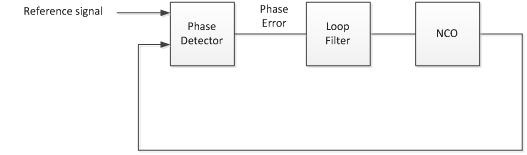Figure 1.1  Digital PLL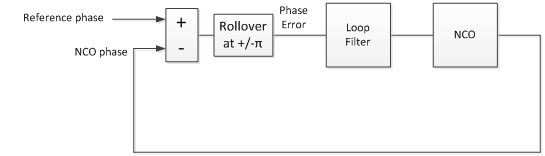Figure 1.2  Digital PLL model using phase signals

## 2. Components of the DPLL Time domain model

As shown in Figure 1.2, the DPLL contains an NCO, phase detector, and a loop filter.  We now describe these blocks for a 2nd order PLL [1, 2].  To keep things simple, all blocks use the same sample frequency.  This discussion deals with the phase of sampled sine waves.  For background, see Appendix B.

### The NCO

The phase of a sine wave is given by:

```phi= 2*pi*mod(f*n*Ts,1)
```

Here f is the frequency, n is sample index, and Ts is sample time.  To create an NCO, we just implement the above equation.  But rather than generate phase in radians, we remove the 2*pi to give phase in cycles.  Then the range of phase (renamed u) is 0 to 1.

```u = mod(f*n*Ts,1);      % cycles
```

The implementation in Figure 2.1 is an accumulator that rolls over when x >1, with input f*Ts = f/fs.  The output u is phase in cycles.  The output y is a sine wave at frequency = f.  The cosine function is generated using a lookup table or CORDIC algorithm.

For our DPLL we make two modifications, as shown in Figure 2.2.  We add input Vtune and scaling Knco to allow an offset from the center frequency, f.  Typically, Knco << 1.  Also, we removed the sinusoidal output; only the phase output is needed in the model.  With the sine output removed, we refer to the NCO as a phase accumulator.

The difference equations for the phase accumulator are simply:

```x = f*Ts + u(n-1) + vtune(n-1)*Knco;     % cycles NCO phase
u(n) = mod(x,1);                         % cycles NCO phase mod 1
```

A note on quantization:  In a digital implementation, u must be quantized to a reasonable number of bits.  For simplicity, we have not included quantization in this and subsequent models, but it can easily be added.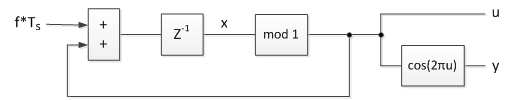Figure 2.1.  Basic NCO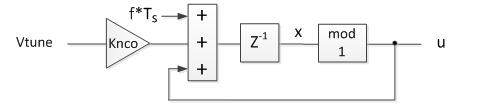Figure 2.2.  Phase Accumulator for DPLL.   u = phase in cycles.

### The Phase Detector

The phase detector is shown in figure 2.3.  It performs a difference, then a rollover function when the phase crosses +/- ½ cycle (+/- π radians).  The difference equations are:

```pe= ref_phase(n-1) - u(n-1);     % phase error
pe= 2*(mod(pe+1/2,1) - 1/2);     % wrap if phase crosses +/- 1/2 cycle
phase_error(n) = pe;
```

The output of the phase detector has a range of +/-1 for an input phase difference of 1 cycle.  Thus the phase detector gain is:

Kp = 2     in units of cycle-1.

A plot of phase detector output vs. phase error is shown in Figure 2.4.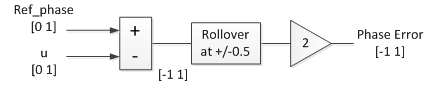Figure 2.3  Phase Detector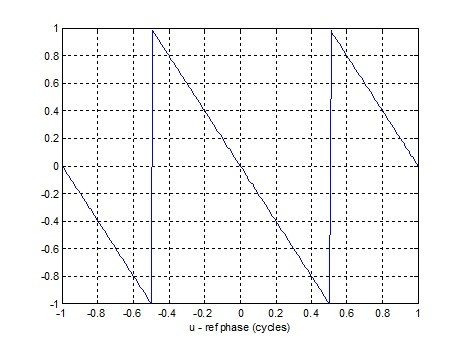Figure 2.4  Phase Detector Characteristic

### The Loop Filter

For a 2nd order loop, the loop filter consists of a proportional gain KL summed with an integrator having gain KI.  It is called a Proportional + Integral or Lead-Lag filter.  The difference equations are:

```int(n) = KI*pe + int(n-1);     % integrator
vtune(n) = int(n) + KL*pe;     % loop filter output
```

where pe is the phase error.  KL and KI determine the damping and natural frequency of the PLL.  We will show how to calculate KL and KI in Part 2.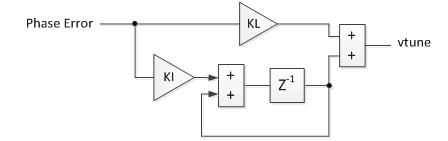Figure 2.5  Loop Filter

Putting all the components together, the DPLL time domain model is shown in Figure 2.6.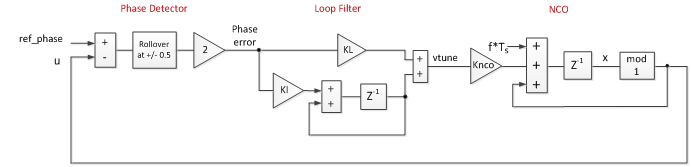Figure 2.6  DPLL Time Domain Model Block Diagram

## 3. Example Cases of DPLL Time Domain model

The Matlab script for the time domain DPLL is listed in Appendix A.  The input parameters for the examples are:

fs                                                    25 MHz
Reference frequency                     8 MHz
Initial reference phase                   0.7 cycles
NCO initial frequency error           -100 ppm or -500 ppm (-800 or -4000 Hz)
Knco                                                1/4096
N                                                    30,000 or 100,000 samples
fn                                                    5 kHz or 400 Hz   loop natural frequency.  fn = ωn/(2π)
ζ (zeta)                                           1.0                            loop damping coefficient
KL, KI                                               calculate from fn and ζ

The first example has initial frequency error less than loop natural frequency, and the second example has initial frequency error much greater than loop natural frequency.  The formulas for KL and KI to obtain the desired natural frequency and damping will be covered in Part 2.

Example 1.  Initial frequency error < Loop natural frequency.  Loop natural frequency = 5 kHz and NCO initial frequency error = -800 Hz.

For this case, the loop behaves as a linear system.  Note we have allowed Vtune to exceed [-1 1], rather than clipping at that level.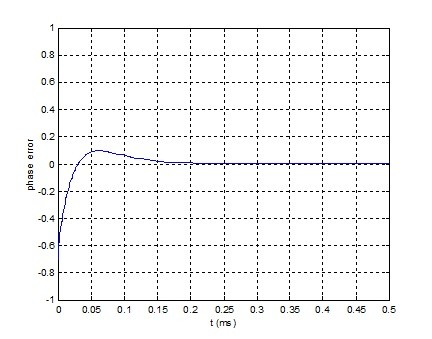Figure 3.1    Phase error.

NCO Initial freq error = -800 Hz.  Initial ref phase = 0.7 cycles.  fn = 5 kHz, ζ = 1.0.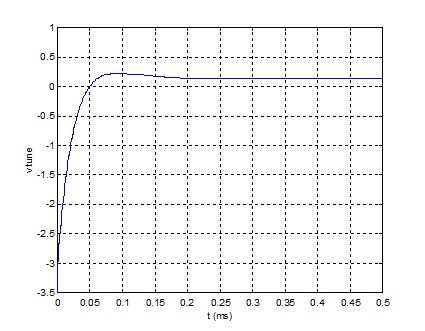Figure 3.2    Vtune

NCO Initial freq error = -800 Hz.  Initial ref phase = 0.7 cycles.  fn = 5 kHz, ζ = 1.0.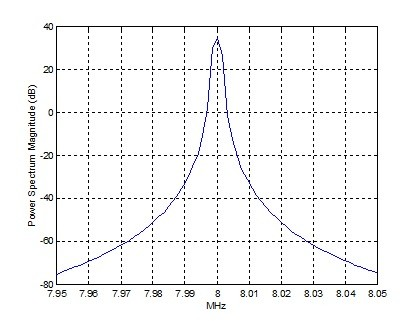Figure 3.3    Output Spectrum (bin spacing = 25E6/2^14 = 1.53 kHz)

Example 2.  Initial frequency error >> Loop natural frequency.  Loop natural frequency = 400 Hz and NCO initial frequency error = -4 kHz.

For this case, the phase detector repeatedly wraps due to the large initial frequency error.  Thus the loop is highly non-linear during acquisition.  Note the time scales of the plots are longer than for the previous example.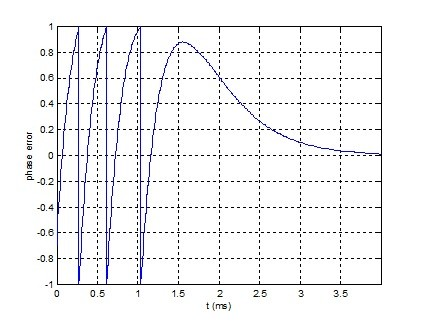Figure 3.4    Phase error

NCO Initial freq error = -4 kHz.  Initial ref phase = 0.7 cycles.  fn = 400 Hz, ζ = 1.0.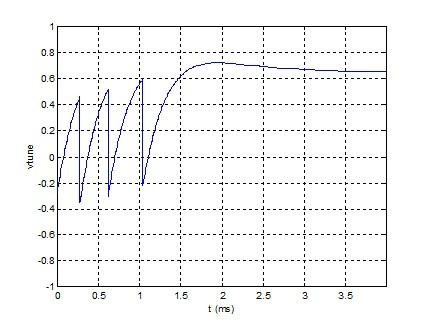Figure 3.5    Vtune

NCO Initial freq error = -4 kHz.  Initial ref phase = 0.7 cycles.  fn = 400 Hz, ζ = 1.0.

### Appendix A.  Time domain model of DPLL with fn = 5 kHz

```% pll_time2.m 6/3/16 nr
% Digital PLL model in time using difference equations.
% fn = 5 kHz NCO initital freq error = -100ppm*8 MHz = -800 Hz
N= 30000;                   % number of samples
fref = 8e6;                 % Hz freq of ref signal
fs= 25e6;                   % Hz sample rate
Ts = 1/fs;                  % s sample time
n= 0:N-1;                   % time index
t= n*Ts*1000                % ms
init_phase = 0.7;           % cycles initial phase of reference signal
ref_phase = fref*n*Ts + init_phase;     % cycles phase of reference signal
ref_phase = mod(ref_phase,1);           % cycles phase mod 1
Knco= 1/4096;                           % NCO gain constant
KI= .0032;                              % loop filter integrator gain
KL= 5.1;                         % loop filter linear (proportional) gain
fnco = fref*(1-100e-6);          % Hz NCO initial frequency
u(1) = 0;
int(1)= 0;
phase_error(1) = -init_phase;
vtune(1) = -init_phase*KL;
% compute difference equations
for n= 2:N;
% NCO
x = fnco*Ts + u(n-1) + vtune(n-1)*Knco;     % cycles NCO phase
u(n) = mod(x,1);                            % cycles NCO phase mod 1
s = sin(2*pi*u(n-1));                       % NCO sine output
y(n)= round(2^15*s)/2^15;                   % quantized sine output
% Phase Detector
pe= ref_phase(n-1) - u(n-1);                % phase error
pe= 2*(mod(pe+1/2,1) - 1/2);             % wrap if phase crosses +/- 1/2 cycle
phase_error(n) = pe;
% Loop Filter
int(n) = KI*pe + int(n-1);                 % integrator
vtune(n) = int(n) + KL*pe;                 % loop filter output
end
plot(t,phase_error),grid
axis([0 0.5 -1 1])
xlabel('t (ms)'),ylabel('phase error'),figure
plot(t,vtune),grid
axis([0 0.5 -3.5 1])
xlabel('t (ms)'),ylabel('vtune'),figure
psd(y(11000:end),2^14,fs/1e6)
axis([7.95 8.05 -80 40]),xlabel('MHz')
```

### Appendix B.    Phase of a sampled sine wave

For a continuous sine wave y = cos(2πft), the phase is 2πft radians.  For a sampled sine wave, we make the following definitions:

```N= 50;         % number of samples
fs= 25e6;      % Hz sample frequency
Ts= 1/fs;      % s sample time
f= 1e6;        % Hz sinewave frequency
```

Noting that 2πf*t  = 2πf*nTs,  the phase is:

```n= 0:N-1;             % sample index
```

To make the phase wrap at phi = 2π, we modify this expression using the modulus function:

```phi= 2*pi*mod(f*n*Ts,1);
```

The phase and its cosine are plotted here: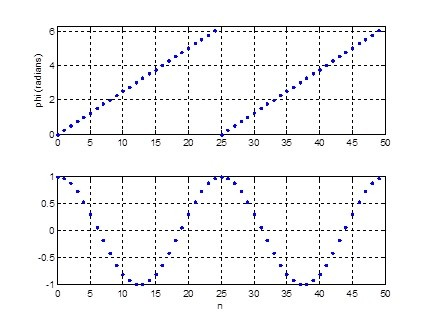Top:  phase phi of sampled cosine wave (radians).

Bottom:  cos(phi)

### References

1.  Gardner, Floyd M., Phaselock Techniques, 3rd Ed., Wiley-Interscience, 2005, Chapter 4.

2.  Rice, Michael, Digital Communications, a Discrete-Time Approach, Pearson Prentice Hall, 2009, Appendix C.

6/3/2016    Neil Robertson

[ - ]
Comment by June 10, 2016This is a very timely post for me. I am currently working on synchronization in communication receivers where digital pll plays a very significant role. I am waiting for the next part, i hope the concepts of acquisition range and time are discussed for a digital PLL which would be very helpful. Thanks...
[ - ]
Comment by June 10, 2016Avi,
In my experience of QAM receivers, it is typically possible for the clock recovery loop to lock without any frequency acquisition aids. This is because the frequency error is not so large compared to the loop bandwidth. On the other hand, the carrier loop typically requires an acquisition aid, such as sweeping.
One interesting point made by Gardner in section 8.3 of his book "If the loop filter contains a perfect integrator, pull-in will be accomplished no matter how large the initial frequency error. (This statement neglects clipping limits; the loop clearly cannot pull in a signal that requires excessive control voltage to the VCO)."

This applies to the digital PLL's we are discussing. As a practical matter though, it can take a loop too long to acquire.
[ - ]
Comment by July 20, 2016Abiya,

Thanks. I hope you like part 2 of the article.
[ - ]
Comment by August 15, 2016I found both Parts 1 and 2 short, to the point, and very valuable. I own the Floyd Gardner book - this information augments the information in the book very well. I learn best when I either have to code up algorithms myself or can see what others have done, allowing me to "play around" with the various components to gain insights into the functionality. MATLAB was a very good choice. Thanks, Neil.
[ - ]
Comment by August 15, 2016Hi, glad you liked it. Let me know if there are any other DPLL topics you'd like to see addressed.
regards, Neil
[ - ]
Comment by June 23, 2017Hi Neil,

I have a question regarding the Loop Filter. Appendix A loop filter calculations do not seem to account for the delay element in the lower path of loop filter. As per the Figure 2.6, KI*pe should arrive one sample later than KL*pe at the input to adder. Could you please explain.

% Loop Filter
int(n) = KI*pe + int(n-1); % integrator
vtune(n) = int(n) + KL*pe; % loop filter output

[ - ]
Comment by June 27, 2017Hi,

Yes, I think you are right.  I think I should have used the previous sample of pe.  It turns out that a delay of one sample has a minute effect on the time response because the sample rate is much higher than the loop bandwidth.

regards,

Neil

[ - ]
Comment by March 14, 2018Dear,

I'm study the DPLL from your document. I have the Gardner book but I have not the Digital Communications and I want to understand this 3 concept:

1) why you use a rollover on the phase detector? What is its function?

2) why you define the phase in cycle/s?

3) where I find the explanation about your conversion from Laplace transform to z-domain?

[ - ]
Comment by March 14, 2018Hi mosx,

1.  The nature of phase is that it repeats every 1 cycle or 2pi radians.  The inputs to the phase detector roll over every +/- pi radians.  For example, the NCO phase output goes from 0 to 2pi (0 to 1 cycles), then it rolls over.  Since this is the case, there is no point in having the phase detector output range exceed +/- pi.

2.  Using phase in cycles is convenient, because the phase range is then +/-1, which works well for digital implementation.  But it is not necessary to do it this way, you could also use radians.

3.  I guess you mean s --> (z-1)/Ts.  I discuss this in part 2, Appendix C, but I'm not very rigorous.  Another way to look at it:  a discrete-time integrator has H(z) = Ts/(z-1), so you can then claim that 1/s --> Ts/(z-1).  You may want to find a textbook explanation of a discrete integrator in the Z domain.

regards,

Neil

[ - ]
Comment by March 14, 2018Neil

[ - ]
Comment by October 29, 2020Hi neil,

Could you provide a more insight on how you got to the exact formula for roll over?

pe= 2*(mod(pe+1/2,1) - 1/2);

Also if suppose, pe = 0.4 then why is it being rolled off to 0.8 according to your formula?

[ - ]
Comment by October 29, 2020machx5,

The formula implements the block diagram in Figure 2.3.  Maybe a clearer way to do it is as follows, where pe is the NCO phase minus the reference phase.  For this example, pe is a ramp with range of [-1 1] cycles.

```x= -1:.01:1;
pe= -x;              % phase error, cycles
% rollover so range of pe = [-.5 .5] cycles
i= find(pe>=.5);
pe(i)= pe(i)-1;      % wrap phase
k= find(pe<-.5);
pe(k)= pe(k)+1;      % wrap phase
y= 2*pe;             % scale to range of [-1 1]
plot(x,y)
```

For a digital implementation using 2's complement arithmetic, the rollover happens automatically in the adder of Figure 2.3, as long as the number of bits in the adder is correct and the adder does not use clipping logic.

regards,

Neil

[ - ]
Comment by October 21, 2022Hi Neil, great articles.  I'm going through them as I try to implement a DPLL in an FPGA.

I'm still not 100% clear on your explanation 1. above.  Can you please clarify what you mean that the inputs to the phase detector roll over every +/- pi radians? This is confusing to me, as I see them wrapping at 1 (they go from [0,1]-a full-cycle, 2pi) --so I must not be understanding what you mean.

I understand how phase repeats every 2pi radians, but I'm struggling to follow the statement, " Since this is the case, there is no point in having the phase detector output range exceed +/- pi."

Also, if the ref signal is 0.9. and the NCO is at 0.1 cycles, then the phase difference is 0.8.  The phase error is then given as -0.4 --but, shouldn't it be -0.8?

Jorge

[ - ]
Comment by October 21, 2022Hi Jorge,

Keep in mind that I am performing all calculations in cycles, not radians.

The phase detector rolls over at +/- 0.5 cycles.  Take a look at the alternative Matlab code in my comment above from Oct 29,2020.  Also, note that there is a gain of 2 after the rollover function, which gives output range of +/-1.

regards,

Neil

[ - ]
Comment by October 21, 2022Hi Neil,

thanks for your prompt response!  Yes, I am aware that everything is done in cycles....but I was alluding to your statement that was referring to signal in radians, so I didn't want to confuse the issue.  I get the conversion....

Is the 0.5cycles for the roll-over  an arbitrary selection? Are the inputs to the detector rolling over at 0.5cycles because you "made it that way"?

Thanks again,

Jorge

Thanks again,
jorge

[ - ]
Comment by October 21, 2022Jorge,

The input range for rollover should be 1 cycle.  As far as the center point goes, I have not played with this for a few years, so I better not try to guess an answer.  Maybe you can try different values in your model.

Cheers,

Neil

[ - ]
Comment by November 25, 2018Thanks for this serie on DPLL. I struggled alot in finding a matlab implementation with good explanation axed towards real discrete hardware implementation. Much appreciated.

[ - ]
Comment by November 25, 2018DjiBy,

You are welcome!

Neil

[ - ]
Comment by November 28, 2018Hi again Neil,

I am working on implementing a DPLL in my FPGA starting from your blog series here. I went through part 1 implementation in matlab and I have a simple question: Why is the roll over part of the phase detector necessary? Without the rollover, I do observe kind of a chaotic pattern at the "pe" output instead of a good looking triangle wave. Intuitively, it's probably better in a closed loop system but do you have an exact reason to not use "pe" right away?

Best regards,

JB

[ - ]
Comment by November 28, 2018DjiBy,

If you don't do a defined rollover of the phase error, it will sooner or later hit positive or negative full scale and either saturate or roll-over, depending on the design.  I don't think that will work.

Note that when the PD rolls over at +/- 1/2 cycle (+/- pi radians),its output has a dc component that drives the loop to lock. (See for example ref 1).

regards,

Neil

[ - ]
Comment by November 29, 2018Hi Neil,

Thanks for your answer. Indeed, the most of the frequency content of "pe" after the rollover is near DC, compared to the spectrum before the roll over. If I understand correctly, since the loop filter is limited in term of bandwidth, that kind of signal is preferred for the DPLL to lock and maintain a stable system?

[ - ]
Comment by November 29, 2018DjiBy,

During acquisition, the shape of the spectral content is not of concern, as long as the PLL is driven to lock.  If the frequency error is large with respect to loop bandwidth, the dc component at the PD output will change slowly, and it will take the loop a long time to lock.

After lock, the PD time-domain output should be zero, with an occasional pulse up or down to maintain lock.  This pulse is then integrated by the loop filter to maintain the correct tuning "voltage".

In the frequency domain, higher frequency noise on the PD output is attenuated by the loop filter -- As you have probably have seen, an example loop filter frequency response is shown in Figure 6 of Part 2 of this series.

regards,

Neil

[ - ]
Comment by May 7, 2019Hi Neil, thanks a lot for your post and great work! After going through it I've got couple of questions and would appreciate if you would be able to answer:

- first question is about terminology: in the integrator part of the loop filter you use 1-st order IIR with the pole at the unit circle. Is it not a generator rather than a filter?

-second question is what modifications to your block diagram will be required if we have to work with sinusoidal waveforms instead of their phases? Should we consider to add some sort of LPF in FD part to get rid of double frequency of the product or loop filter can take care of it?

Thanks!

[ - ]
Comment by May 8, 2019Hello alexLV,

The pole of the integrator is at z = 1 +j0.  By definition, z = exp(jw), where w = 2pi*f/fs.  For z = 1 + j0, w= 0.  So the pole of an integrator, although on the unit circle, occurs at w = 0. So it is not an oscillator.

Take a look at Part 3 of this series that covers locking a PLL to a sinewave:

The design uses a phase detector that has zero output when the loop is locked.  No additional filtering is required.  For some PD's, you may need a LPF, but keep in mind that any LPF is part of the loop and increases the loop order.  This can lead to instability.

regards,

Neil

[ - ]
Comment by August 6, 2020I did enjoy your post. Great tutorial Neil. Please keep it up.

[ - ]
Comment by August 6, 2020Thanks!

To post reply to a comment, click on the 'reply' button attached to each comment. To post a new comment (not a reply to a comment) check out the 'Write a Comment' tab at the top of the comments.